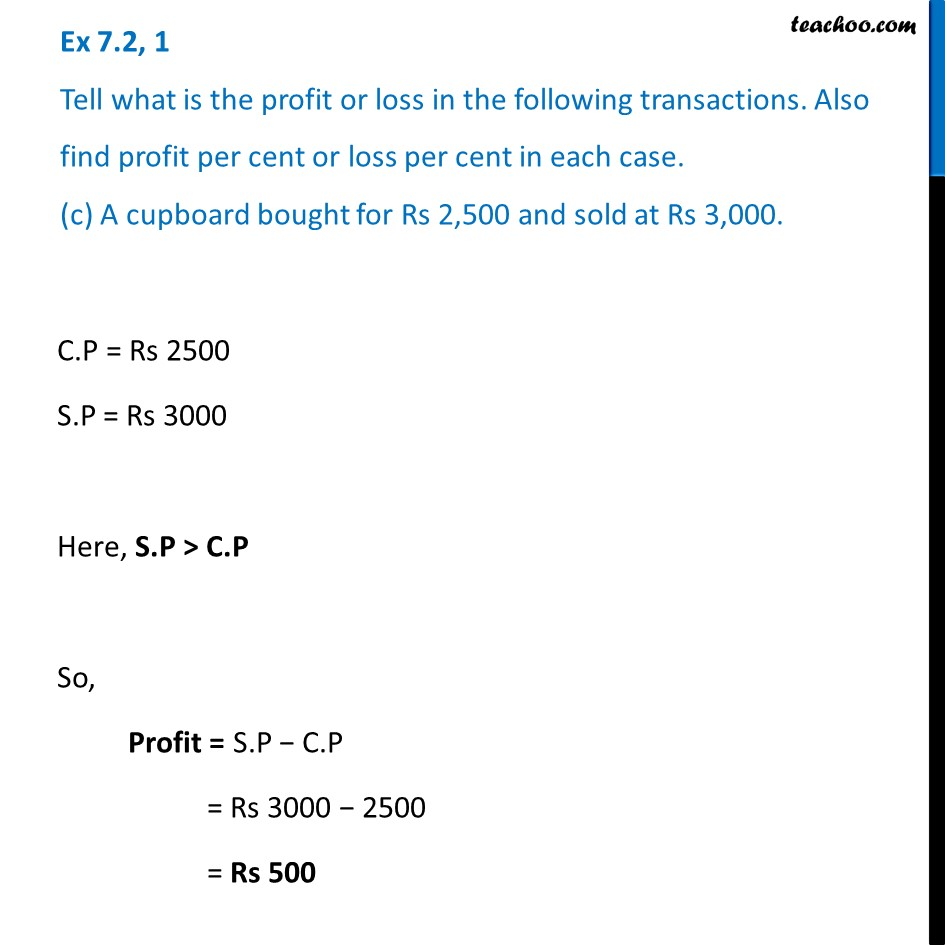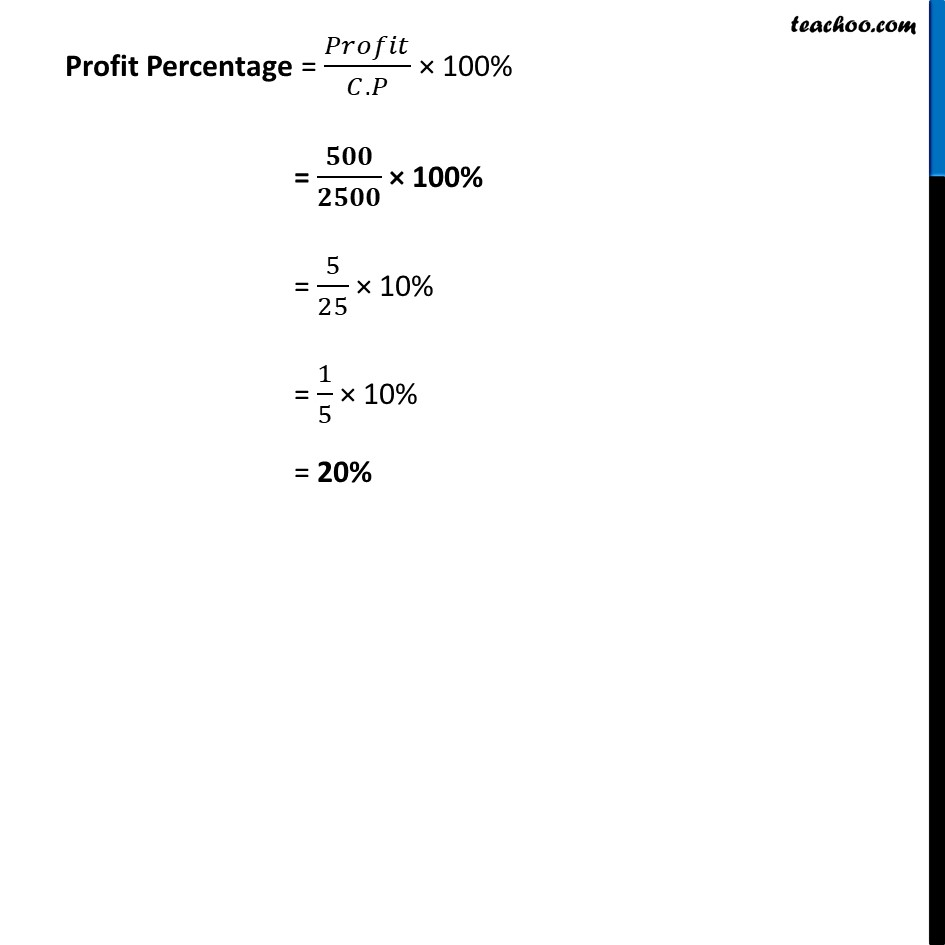Ex 7.2

Chapter 7 Class 7 Comparing Quantities
Serial order wiseLearn in your speed, with individual attention - Teachoo Maths 1-on-1 Class

### Transcript

Ex 7.2, 1 Tell what is the profit or loss in the following transactions. Also find profit per cent or loss per cent in each case. (c) A cupboard bought for Rs 2,500 and sold at Rs 3,000. C.P = Rs 2500 S.P = Rs 3000 Here, S.P > C.P So, Profit = S.P − C.P = Rs 3000 − 2500 = Rs 500 Profit Percentage = 𝑃𝑟𝑜𝑓𝑖𝑡/(𝐶.𝑃) × 100% = 𝟓𝟎𝟎/𝟐𝟓𝟎𝟎 × 100% = 5/25 × 10% = 1/5 × 10% = 20%